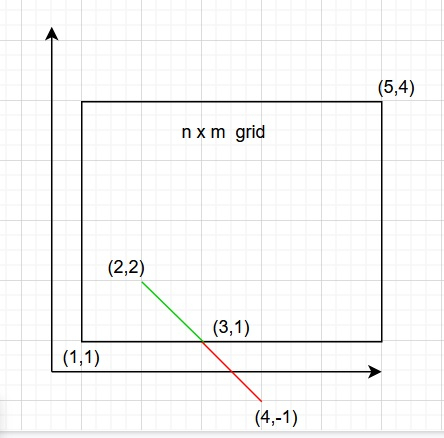# Count possible moves in the given direction in a grid in C++

We are two variables n and m representing a grid of size n x m and initial point x,y to start from.

Also given pairs of steps/moves that can be taken to traverse inside the grid as moves ( (1,1), (2,2) ) etc. Each pair of moves represents the unit of steps taken in the x,y axis. The goal is to find the total steps that can be taken to traverse inside the grid within boundaries [1,n] X [1,m] If n is 5 and m is 4 and current position is 2,2 and step chosen is (1,-1) then taking this step once will lead to (3,1), taking this step again will lead to (4,-1) which is not valid as -1 is out of bound.Let us understand with examples

Input − A = 3, B = 4, x = 1, y = 1; moves = { 1, 1 }, { 0, -1 }

Output − Count of possible moves in the given direction in a grid are − 4

Explanation

Choosing move {1,1} = → (2,2) → (3,3) - 3 steps
Choosing move {0,-1} = → (3,2) → (3,1) - 2 steps
Total 4 steps.

Input − A = 4, B = 4, x =2, y = 2; moves = { 2, 1 }, { -2, -3 }

Output − Count of possible moves in the given direction in a grid are − 1

Explanation

Choosing move {2,1} = → (4,3) - 1 step1
Choosing move {-2,-3} = → (2,0) X out of bound
Total 1 step

## Approach used in the below program is as follows

In this approach we will create a vector representing steps as pair<int,int>. Start traversing from point x,y. Choose a step from the vector and choose the minimum of the value taken in both directions (x axis and y axis). The minimum chosen will allow more moves to take. To move in particular direction, if the cur position x ( or y ) is > n (or m) then the number of moves to reach n (or m) is ( n - cur position )/x. If it is less then the number of moves to reach 1 is ( cur position - 1 )/x.

• Take variables A,B for representing an AXB grid and x,y for starting point.

• Take a vector containing integer pairs as moves (vector <pair<int, int> > ).

• Function possible_moves(int x, int y, int A, int B, vector<pair<int, int>> move, int size) takes all variables and moves and returns the count of possible moves in the given direction in a grid.

• Function possible(int x, int temp_x, int A) takes current position of coordinate as x, corresponding coordinate value in the move as temp_x and limit of Grid for that coordinate as A.

• Now if temp_x is 0 return INT_MAX to make return value as maximum.

• If temp_x is > 0 then moves to reach A will be | A-x |/temp_x

• Else for moving towards 1 moves will be | x-1 |/temp_x.

• Return corresponding calculated moves.

• Inside possible_moves(), take the initial count as 0.

• Traverse vector using for loop from i=0 to i<size.

• Extract coordinates from the current pair of move as temp_x=move[i].first and temp_y=move[i].second.

• Take variable checks as minimum of possible moves using function possible().

• The minimum value in check will be added to count for total steps.

• Now that we chose check, update x and y by check.

• At last we will get the total count of possible moves in the given direction in a grid

• Return count as result.

## Example

Live Demo

#include <bits/stdc++.h>
using namespace std;
int possible(int x, int temp_x, int A){
if(temp_x == 0){
return INT_MAX;
}
if (temp_x > 0){
return abs((A - x) / temp_x);
}
else{
return abs((x - 1) / temp_x);
}
}
int possible_moves(int x, int y, int A, int B, vector<pair<int, int>> move, int size){
int count = 0;
for (int i = 0; i < size; i++){
int temp_x = move[i].first;
int temp_y = move[i].second;
int check = min(possible(x, temp_x, A), possible(y, temp_y, B));
count = count + check;
x = x + check * temp_x;
y = y + check * temp_y;
}
return count;
}
int main(){
int A = 3, B = 6, x = 3, y = 3;
vector<pair<int, int> > move = {
{ 2, -1 },
{ 0, 1 },
{ 1, -2 }
};
int size = move.size();
cout<<"Count of possible moves in the given direction in a grid are: "<<possible_moves(x, y, A, B, move, size);
return 0;
}

## Output

If we run the above code it will generate the following output −

Count of possible moves in the given direction in a grid are: 3

Updated on: 02-Dec-2020

153 Views